## Exercises

1. Forward projections in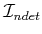:
1. Starting from a nondeterministic I-state,, and applying an action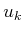, derive an expression for the nondeterministic one-stage forward projection by extending the presentation in Section 10.1.2.
2. Determine an expression for the two-stage forward projection starting fromand applyingand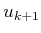.
2. Forward projections in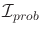:
1. Starting from a probabilistic I-state,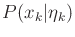, and applying an action, derive an expression for the probabilistic one-stage forward projection.
2. Determine an expression for the two-stage forward projection starting fromand applyingand.
3. Determine the strong and weak backprojections on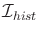for a given history I-state,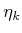. These should give sets of possible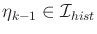.
4. At the end of Section 11.3.2, it was mentioned that an equivalent DFA can be constructed from an NFA.
1. Give an explicit DFA that accepts the same set of strings as the NFA in Figure 11.8b.
2. Express the problem of determining whether the NFA in Figure 11.8b accepts any strings as a planning problem using Formulation 2.1.
5. This problem involves computing probabilistic I-states for Example 11.14. Let the initial I-state be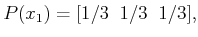(11.90)

in which theth entry in the vector indicates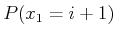. Let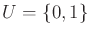. For each action, a state transition matrix can be specified, which gives the probabilities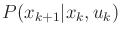. For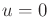, let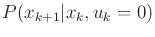be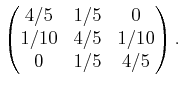(11.91)

The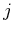th entry of theth row yields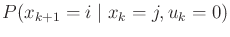. For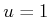, let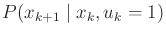be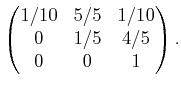(11.92)

The sensing model is specified by three vectors: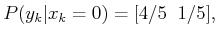(11.93)(11.94)

and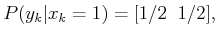(11.95)

in which theth component yields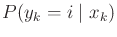. Suppose that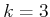and the history I-state obtained so far is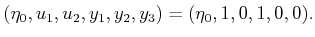(11.96)

The task is to compute the probabilistic I-state. Starting from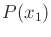, compute the following distributions: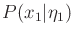,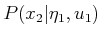,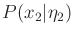,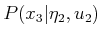,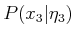.

6. Explain why it is not possible to reach every nondeterministic I-state from every other one for Example 11.7. Give an example of a nondeterministic I-state that cannot be reached from the initial I-state. Completely characterize the reachability of nondeterministic I-states from all possible initial conditions.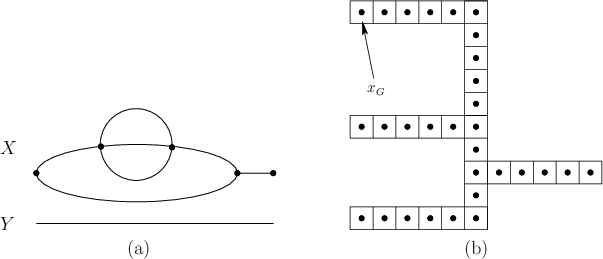7. In the same spirit as Example 11.21, consider a point moving on the topological graph shown in Figure 11.33. Fully characterize the connectivity of(you may exploit symmetries to simplify the answer).
8. Design an I-map for Example 11.17 that is not necessarily sufficient but leads to a solution plan defined over only three derived I-states.
9. Consider the discrete problem in Figure 11.33b, using the same sensing and motion model as in Example 11.17.
1. Develop a sufficient I-map and a solution plan that uses as few derived I-states as possible.
2. Develop an I-map that is not necessarily sufficient, and a solution plan that uses as few derived I-states as possible.
10. Suppose that there are two I-maps,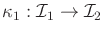and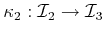, and it is given that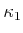is sufficient with respect to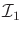, andis sufficient with respect to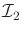. Determine whether the I-map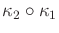is sufficient with respect to, and prove your claim.
11. Propose a solution to Example 11.16 that uses fewer nondeterministic I-states.
12. Suppose that a point robot moves in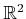and receives observations from three homing beacons that are not collinear and originate from known locations. Assume that the robot can calibrate the three observations on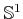.
1. Prove that the robot can always recover its position in.
2. What can the robot infer if there are only two beacons?
13. Nondeterministic I-state problems:
1. Prove that the nondeterministic I-states for Example 11.23 are always a single connected region whose boundary is composed only of circular arcs and line segments.
2. Design an algorithm for efficiently computing the nondeterministic I-states from stage to stage.
14. Design an algorithm that takes as input a simply connected rectilinear region (i.e., described by a polygon that has all right angles) and a goal state, and designs a sequence of tray tilts that guarantees the ball will come to rest at the goal. Example 11.24 provides an illustration.
15. Extend the game-theoretic formulation from Section 11.7.2 of history I-spaces to continuous time.
16. Consider the where did I come from?'' problem.
1. Derive an expression for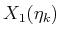.
2. Derive an expression for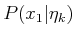.
17. In the game of Example 11.27, could there exist a point in the game at which one player has not yet observed every possible hit'' yet it knows the state of the game (i.e., the exact location of all ships)? Explain.
18. When playing blackjack in casinos, many card-counting strategies involve remembering simple statistics of the cards, rather than the entire history of cards seen so far. Define a game of blackjack and card counting as an example of history I-states and an I-map that dramatically reduces the size of the I-space, and an information-feedback plan.

Implementations

19. Implement the Kalman filter for the case of a robot moving in the plane. Show the confidence ellipsoids obtained during execution. Be careful of numerical issues (see ).
20. Implement probabilistic I-state computations for a point robot moving in a 2D polygonal environment. Compare the efficiency and accuracy of grid-based approximations to particle filtering.
21. Design and implement an algorithm that uses nondeterministic I-states to play a good game of Battleship, as explained in Example 11.27.

Steven M LaValle 2020-08-14